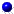Nonequispaced fast Fourier transform
Summation on the sphere
Fast summation on the sphere based on NFSFT

# Fast summation on the sphere based on NFSFT

This library of C functions computes approximations of sums of the following form.

Given $$D,L \in N$$, a set of arbitrary source nodes $$\mathcal{Y} :=\{\eta_{l} \in \mathbb{S}^2: l = 0,\dots,L-1\}$$, and a vector of real coefficients $$\mathbf{b}:=(b_{l})_{l=0}^{L-1}$$, evaluate the sum $f(\xi) := \sum_{l = 0}^{L-1} b_{l} K(\eta_{l} \cdot \xi)$ on a set of arbitrary target nodes $$\mathcal{X} := \{\xi_{d} \in \mathbb{S}^2: d=0,\dots,D-1\}$$. Here $$K$$ is a kernel function. Such kernels include the Abel-Poisson and singularity kernels as well as locally supported kernels and the spherical Gaussian kernel.

Our algorithm is based on the fast Fourier transform for nonequispaced nodes on the sphere (NFSFT) and therefore depends on the NFFT C-library. New kernels are easily incorporated by defining an appropriate C-function.

The algorithms are implemented by Jens Keiner and Stefan Kunis in ./applications/fastsumS2. Related paper areKeiner, J., Kunis, S. and Potts, D.
Fast summation of radial functions on the sphere.
Computing 78, 1-15,   (full paper ps, pdf),   2006